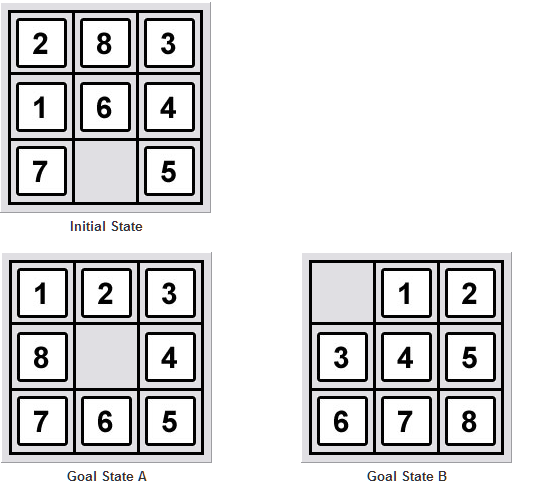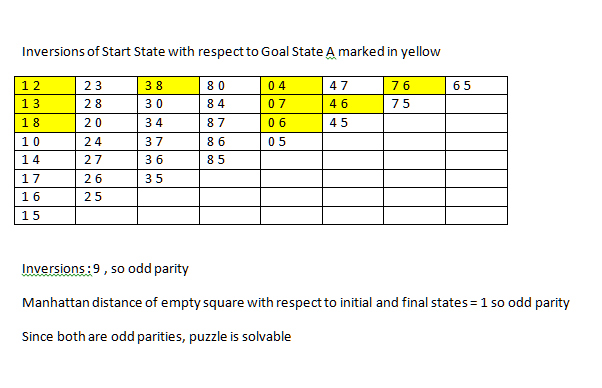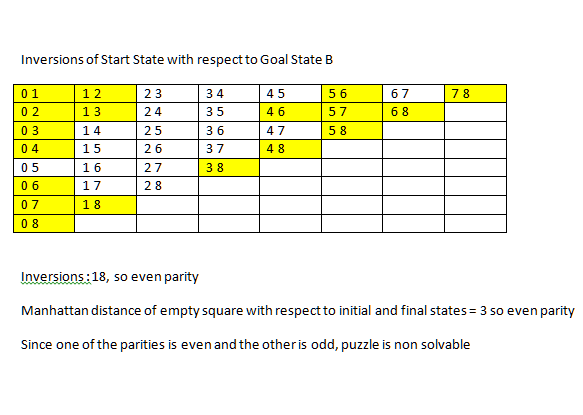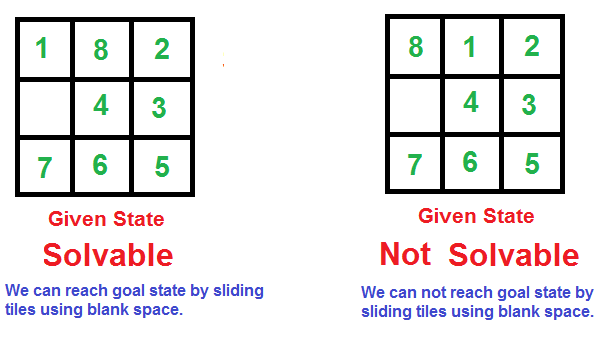2 views

I have built an 8 puzzle solver using Breadth First Search. I would now want to modify the code to use heuristics. I would be grateful if someone could answer the following two questions:

Solvability

How do we decide whether an 8 puzzle is solvable? (given a starting state and a goal state ) This is what Wikipedia says:

The invariant is the parity of the permutation of all 16 squares plus the parity of the taxicab distance (number of rows plus the number of columns) of the empty square from the lower right corner.

Unfortunately, I couldn't understand what that meant. It was a bit complicated to understand. Can someone explain it in a simpler language?

Shortest Solution

Given a heuristic, is it guaranteed to give the shortest solution using the A* algorithm? To be more specific, will the first node in the open list always have a depth ( or the number of movements made so fat ) which is the minimum of the depths of all the nodes present in the open list?

Should the heuristic satisfy some condition for the above statement to be true?

Edit: How is it that an admissible heuristic will always provide the optimal solution? And how do we test whether a heuristic is admissible?

I would be using the heuristics listed here

Manhattan Distance Linear Conflict Pattern Database Misplaced Tiles Nilsson's Sequence Score N-MaxSwap X-Y Tiles out of row and column

For clarification from Eyal Schneider :by (108k points)

How to find if a given state is solvable?

Following are two examples, the first example can reach the goal state by a series of slides. The second example cannot.Following is a simple rule to check if an 8 puzzle is solvable.

It is not possible to solve an instance of 8 puzzles if a number of inversions are odd in the input state. In the examples given in the above figure, the first example has 10 inversions, therefore solvable. The second example has 11 inversions, therefore unsolvable.

What is an inversion?

A pair of tiles form an inversion if the values on tiles are in reverse order of their appearance in goal state. For example, the following instance of 8 puzzles has two inversions, (8, 6) and (8, 7).

1   2 3

4   _ 5

8   6 7

And about the shortest solution, the A* tree search algorithm is guaranteed to be optimal (i.e., find the shortest path if one exists) if the heuristic is admissible (i.e., never overestimates the path cost to the nearest goal). Formally, a heuristic 'h' is admissible if for every node n, 0 <= h(n) <= h*(n), where h*(n) is the exact cost of reaching a nearest goal from n.

However, A* tree search may end up doing exponentially more repeated work. We need a way to close off expanded nodes so that they are not expanded again, resulting in a graph search algorithm. A* graph search is optimal only if the heuristic used is consistent i.e., it estimates the path cost incrementally so the heuristic value along a path never decreases. Formally, a heuristic 'h' is consistent if for every node n and for every successor s of n, h(n) <= h(p) + cost(n, p).

Manhattan distance is a consistent heuristic for the 8-puzzle problem and A* graph search, equipped with Manhattan distance as a heuristic, will indeed find the shortest solution if one exists.

There is a written detailed explanation of A* search and provided python implementation of N-puzzle problem using A* here: A* search explanation and N-puzzle python implementation